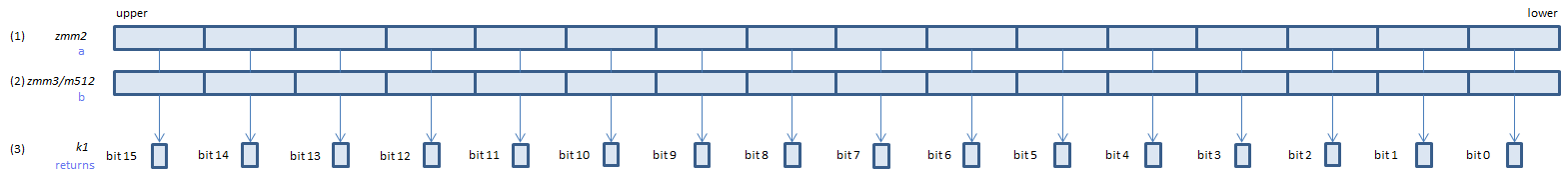﻿ cmpordps

## CMPORDPS - CoMPare ORDered Packed Single

CMPORDPS xmm1, xmm2/m128    (S1
__m128 _mm_cmpord_ps(__m128 a, __m128 b)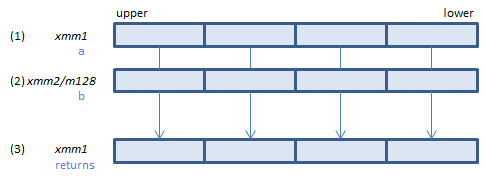For each float, if neither of (1)(2) is NaN, set 1, else set 0, to all bits of the corresponding float of (3).
VCMPORDPS xmm1, xmm2, xmm3/m128    (V1
__m128 _mm_cmpord_ps(__m128 a, __m128 b)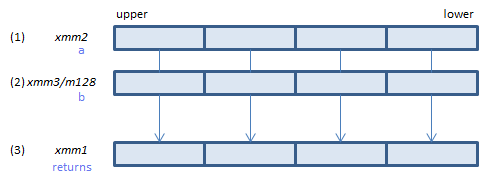For each float, if neither of (1)(2) is NaN, set 1, else set 0, to all bits of the corresponding float of (3).
VCMPORDPS ymm1, ymm2, ymm3/m256    (V1
__m256 _mm256_cmp_ps(__m256 a, __m256 b, _CMP_ORD_Q)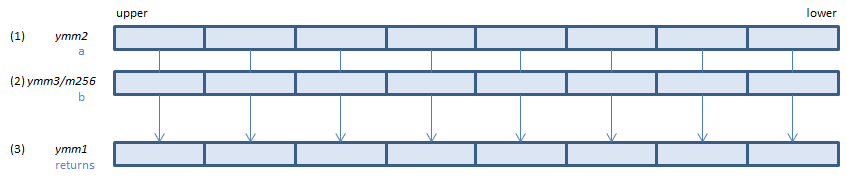For each float, if neither of (1)(2) is NaN, set 1, else set 0, to all bits of the corresponding float of (3).
VCMPORDPS k1{k2}, xmm2, xmm3/m128/m32bcst    (V5+VL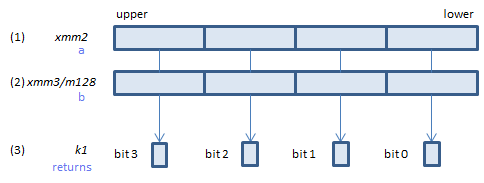For each float, if neither of (1)(2) is NaN, set 1, else set 0, to the corresponding bit of (3).
If k2 bit is 0, the comparison is not done and the corresponding bit of (3) is set to zero. Upper bits of (3) are zero cleared.
VCMPORDPS k1{k2}, ymm2, ymm3/m256/m32bcst    (V5+VL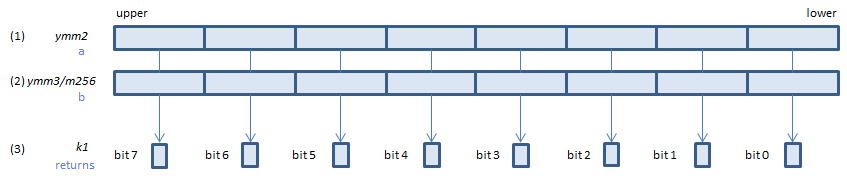For each float, if neither of (1)(2) is NaN, set 1, else set 0, to the corresponding bit of (3).
If k2 bit is 0, the comparison is not done and the corresponding bit of (3) is set to zero. Upper bits of (3) are zero cleared.
VCMPORDPS k1{k2}, zmm2, zmm3/m512/m32bcst{sae}    (V5